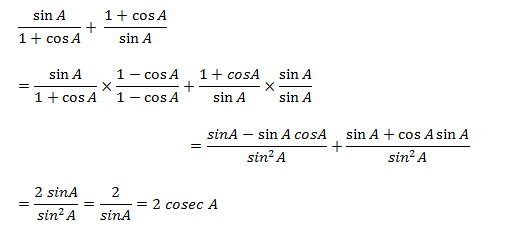# SSC CGL 2018 Practice Test Papers | Quantitative Aptitude (Day-40)

Dear Aspirants, Here we have given the Important SSC CGL Exam 2018 Practice Test Papers. Candidates those who are preparing for SSC CGL 2018 can practice these questions to get more confidence to Crack SSC CGL 2018 Examination.

[WpProQuiz 3342]

Click “Start Quiz” to attend these Questions and view Explanation

1) Simplify: Sin A/(1+cosA)+(1+cosA)/sin A

a) 2 sin A

b) cos A

c) 2 cosec A

d) 2 tan A

2) The average age of 3 children in a family ranges from 2 to 13 years. No two children have same age. The maximum average age of 3 children can be______

a) 12

b) 11

c) 10

d) 6

3) Simplify: 77×335 + 37×665 – 40×335

a) 43000

b) 37000

c) 51000

d) 40000

4) If a= 1×2×3×…….. ×100 and b= 101×102×103×104, then find LCM of a and b

a) 101 ab

b) 103a

c) 10403a

d) 10608a

5) A total of p students in q colleges and in each college the number of students is equal to twice the number of colleges. If 800 < p < 1200 then which of the following is not the possible value of q?

a) 23

b) 25

c) 24

d) 22

6) What is the least natural number which when divided by 20, 18 and 15 leaves remainders of 6, 4 and 1 respectively?

a) 96

b) 126

c) 166

d) 144

7) In a chase, a hare takes 5 leaps for every four leaps of Jackal. If four leaps of hare is equal to five leaps of Jackal, Find the ratio of their speeds.

a) 4:5

b) 16: 25

c) 25: 16

d) 4: 5

8) Sangeetha gave one-fourth of the amount she had to Hema. Hema in turn gave half of what she received to Aparna. If the difference between the remaining amount with Sangeetha and the amount received by Aparna is Rs. 750, how much money did Hema receive from Sangeetha?

a) Rs. 200

b) Rs. 300

c) Rs. 400

d) Rs. 500

9) A father’s present age is seven years less than 30 times his son’s age 20 years ago. Also, the father’s present age is 31 years more than his son’s present age. Find the present age of the son.

a) 20

b) 25

c) 23

d) 22

10) The curved surface area of a sphere is 6π square unit. The volume of the sphere is –

a) 8/3 π cubic unit

b) 8 √3π cubic unit

c) 8√3/5 π cubic unit

d) 8√ 2/3π cubic unitMaximum age of 3 children can be 11, 12 and 13

Sum of their age =11+12+13=36

Average age= 36/3=12

77×335+37×665 – 40×335 = 37×335 + 37×665

= 37 (335+665)

= 37 ×1000

= 37000

a= 1×2×3x…×100 and

b= 101×102×103×104

101 and 103 are prime numbers. And factors of 104 and 108 are in a.

104= 23 x13

108=33x22

Therefore LCM of a and b= 101x103a=10403a

p/q = no. of students in each college

p/q = 2q => p = 2q2

=> 800 < 2q2 < 1200

=> 400 < q2 < 600

Q may be 21, 22, 23, 24.

So the value of q cannot be 25.

Common difference = (20 – 6) = (18 – 4) = (15 – 1) = 14

Required number = (LCM of 20, 18, 15) – common difference = 180 – 14 = 166

Number of leaps taken by Hare = 5

Number of leaps taken by Jackal = 4

4 leaps of Hare = 5 leaps of Jackal

1 leap of Hare = 5/4 leaps of Jackal

• Hare takes 5/4×5 and Jackal takes 4 steps.

Ratio of Speeds= 25/4: 4=25: 16

Let sangeetha’s initial amount = x

Amount Sangeetha gave to Hema = x/4

Remaining amount with Sangeetha = 3x/4

Amount Hema gave to Aparna = x/8

3/4x – 1/8x = 750

x = 1200

Amount received by Hema = x/4 = 1200/4 = 300

Answer: Let the present age of son and father is s and f respectively.

F = 30(s – 20) – 7 —– (i)

F = S + 31 ——- (ii)

S+31 = 30(s – 20) – 7

S = 22

Age of son = 22

Surface area of sphere = 6π

Curved surface area =3πr2= 6π

r =√ 2

So, value of sphere = 4 /3πr3

= 4/3π (√2)3

= 8√ 2/3π cubic unit